Next: Input Cards Up: usermanual Previous: Implementation   Contents

# COMPREFPHA

In this chapter the processing of step COMPREFPHA is described. This step can be performed as soon as the precise orbits are known. The recommended approach is to compute this only after the computation of the interferogram, and then use the step SUBTRREFPHA to subtract it. This step is not required if method "exact" is used in step SUBTRREFPHA.

The flatearth correction (the phase caused by the reference surface (WGS84 for now)) is computed in this step. For a certain (line,pixel) in the master image the corresponding coordinates (x,y,z) of the master and slave satellite and the point P on the reference ellipsoid are computed, utilizing the set of equations (Doppler, range and ellipsoid equation), see annex D. Then the parallel baseline and the phase is computed.

The parallel baseline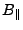is defined as (M/S are positions of master/slave, P is the position of the point on the reference surface):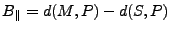(X1)

The phase of a pixel in the master image is defined as: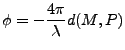(X2)

The reference phase for this pixel is defined as:(X3)

The reference phase is computed in a number of points distributed over the total image in this way, after which a 2d-polynomial is estimated (least squares) fitting these 'observations'. (So a plane can be fitted by setting the degree to 1.)

A 2d-polynomial is defined as: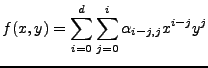(X4)

Thus the order of the coefficients (line,pixel) is (degree d):
d=0:(1)
d=1: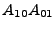(2, 3)
d=2: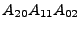(4, 5, 6)
d=3: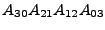(7, 8, 9, 10)

Thus the number of coefficients (unknowns) equals: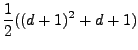(X5)

A polynomial of degree 5 normally is sufficient to model the reference phase for a full scene. A lower degree might be selected for smaller images, which also should increase the stability of the normalmatrix. We would expect the higher order terms to be small because the polynomial describes a smooth, long wave body (ellipsoid). To force the polynomial to be smooth one might consider always using a polynomial of degree 2 or 3.